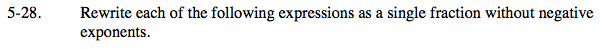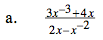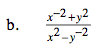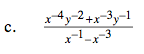### Home > PC > Chapter 5 > Lesson 5.1.2 > Problem5-28

5-28.Multiply by a GIANT ONE representing the highest power to eliminate.

$\left (\frac{3x^{-3}+4x}{2x-x^{-2}} \right ) \left(\frac{x^{3}}{x^{3}} \right )$

$\left (\frac{3+4x^{4}}{2x^{4}-x} \right )$What GIANT ONE should you multiply this fraction by?

$\frac{x^{2}y^{2}}{x^{2}y^{2}}$What GIANT ONE should you multiply this fraction by?

$\frac{x^{4}y^{2}}{x^{4}y^{2}}$